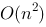# MOOC数据结构 第五周

1.下面关于串的叙述中，正确的是（ A）。
A.串是一种特殊的线性表
B.串中元素只能是字母
C.空串就是空白串
D.串的长度必须大于零
2.两个字符串相等的条件是（D ）。
A.串的长度相等
B.含有相同的字符集
C.都是非空串
D.两个串的长度相等且对应位置的字符相同
3.若串str=“Software”，其子串的个数是（D ）。
A.8
B.9
C.36
D.37
4.一个链串的节点类型定义为
#define NodeSize 6
typedef struct node
{ char data[NodeSize];
struct node next;

A.1/3
B.1/2
C.2/3
D.3/4

1)/(6*1+2)=3/4。
5.串采用节点大小为1的链表作为其存储结构，是指（C ）。
A.链表的长度为1
B.链表中只存放一个字符
C.链表中每个节点的数据域中只存放一个字符
D.以上都不对
6.对于一个链串s，查找第一个字符值为x的算法的时间复杂度为（ B）。
A.O(1)
B.O(n)
C.D.以上都不对
7.设有两个串p和q，其中q是p的子串，则求q在p中首次出现位置的算法称为（C ）。
A.求子串
B.串联接
C.模式匹配
D.求串长
8.在串的简单模式匹配中，当模式串位j与目标串位i比较时，两字符不相等，则i的位移方式是（C ）。
A.i++
B.i=j+1
C.i=i-j+1
D.i=j-i+1
9.在KMP模式匹配中，用next数组存放模式串的部分匹配信息。当模式串位j与目标串位i比较时，两字符不相等，则i的位移方式是（B ）。
A.i=next[j]
B.i不变
C.j不变
D.j=next[j]
10.在KMP模式匹配中，用next数组存放模式串的部分匹配信息。当模式串位j与目标串位i比较时，两字符不相等，则j的位移方式是（D ）。
A.i=next[j]
B.i不变
C.j不变
D.j=next[j]
1.设s为一个长度为n的串，其中的字符各不相同，则s中的互异非平凡子串（非空且不同于s本身）的个数是多少？

2.在KMP算法中，计算模式串的next时，当j=0时，为什么要取next=-1？

3.给出以下模式串的next值和nextval值：
（1）ababaa
（2）abaabaab
(1)
|j | 0 |1 | 2 | 3 | 4 | 5 |
|t[j] | a | b | a | b | a | a |
| next[j] | -1 | 0 | 0 | 1 | 2 | 3 |
| nextval[j] | -1 | 0 | -1 | 0 | -1 | 3 |
(2)
|j | 0 |1 | 2 | 3 | 4 | 5 | 6 | 7 |
|t[j] | a | b | a | a | b | a | a | b |
| next[j] | -1 | 0 | 0 | 1 | 1 | 2 | 3 | 4 |
| nextval[j] | -1 | 0 | -1 | 1 | 0 | -1| 1 | 1 |
4.设有一个顺序串s，其字符仅由数字和小写字母组成。设计一个算法将s中所有数字字符放在前半部分，所有小写字母字符放在后半部分。并给出你所设计的算法的时间和空间复杂度。

#include <iostream>
using namespace std;
const int max=100;
typedef struct
{
char data;
int length;
}SqString;
void f(SqString &s)
{
int i=0,j=s.length-1;
while(i<j)
{
while(i<j&&s.data[i]>='0'&&s.data[i]<='9')
i++;
while(i<j&&s.data[j]>='a'&&s.data[j]<='z')
j--;
if(i<j)
{
char c;
c=s.data[i];
s.data[i]=s.data[j];
s.data[j]=c;
}
}
}
int main()
{
SqString s={"a2cvr25d4e",10};
f(s);
cout<<s.data;
return 0;
}


O(n)=T(m)
5.用带头节点的单链表表示链串，每个节点存放一个字符。设计一个算法求s中最长平台的长度，所谓平台是指连续相同字符。

#include <iostream>
#include <stdlib.h>
using namespace std;
const int max=100;
typedef struct snode
{
char data;
struct snode *next;
}LiString;
void StrAssign(LiString *&s,char cstr[])
{
int i;
LiString *r,*p;
s=(LiString *)malloc(sizeof(LiString));
r=s;
for(i=0;cstr[i]!='\0';i++)
{
p=(LiString *)malloc(sizeof(LiString));
p->data=cstr[i];
p->next=r->next;
r->next=p;
r=p;
}
r->next=NULL;
}
void DisStr(LiString *s)
{
LiString *p=s->next;
while(p!=NULL)
{
cout<<p->data<<" ";
p=p->next;
}
cout<<endl;
}
int f(LiString *&s)
{
LiString *p=s->next;
int len=1,maxlen=0;
while(p->next!=NULL)
{
if(p->data==p->next->data)
{
len++;
if(len>maxlen)
maxlen=len;
}
else len=1;
p=p->next;
}
return maxlen;
}
int main()
{
LiString *a;
char s[]="abcccddddde";
StrAssign(a,s);
DisStr(a);
cout<<f(a);
return 0;
}

11-021625
05-0850310-211810
10-111528
06-1552
11-262万+
03-231187
01-193074
07-2325
07-2677